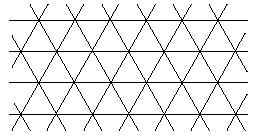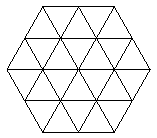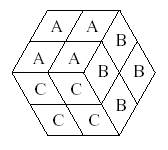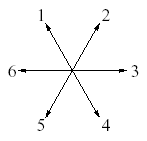Online JudgeProblem SetAuthorsOnline ContestsUser
Web Board
F.A.Qs
Statistical Charts
Problems
Submit Problem
Online Status
Prob.ID:
Register
Authors ranklist
Current Contest
Past Contests
Scheduled Contests
Award Contest
Register
Language:
Rhombs
 Time Limit: 1000MS Memory Limit: 10000K Total Submissions: 202 Accepted: 79

Description

An unbounded triangular grid is a plane covered by equilateral triangles:Two neighboring triangles in the grid form a rhomb. There are 3 types of such rhombs:A grid polygon is a simple polygon which sides consist entirely of sides of triangles in the grid. We say that a grid polygon is rhombastic if it can be partitioned into internally disjoint rhombs of types A, B and C.

As an example let's consider the following grid hexagon:This hexagon can be partitioned into 4 rhombs of type A, 4 rhombs of type B and 4 rhombs of type C:For a given rhombastic grid polygon P compute the numbers of rhombs of types A, B and C in some correct partition.

Write a program that:

• reads a description of a rhombastic grid polygon from the standard input,

• computes the numbers of rhombs of types A, B and C in some correct partition of the polygon,

• writes the results to the standard output.

Input

The first line of the input contains an integer n (3 <= n <= 50 000) - the number of sides of a rhombastic grid polygon. Each of the next n lines contains a description of one side of the polygon. The sides are given one by one in the clockwise order. No two consecutive sides of the polygon lie on the same straight line. The description of a side consists of two integers d and k. Integer d says what is the direction of the side according to the following figure:Integer k is the length of the polygon side measured in the number of sides of grid triangles. Sum of all numbers k is not larger than 100 000.

Process to the end of file.

Output

The first and only line of the output contains three integers separated by single spaces denoting the number of rhombs of type A, B and C respectively, in some partition of the input polygon.

Sample Input

6
1 2
2 2
3 2
4 2
5 2
6 2

Sample Output

4 4 4

Source

[Submit]   [Go Back]   [Status]   [Discuss]Home PageGo BackTo top